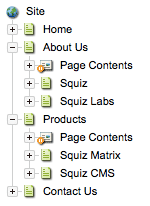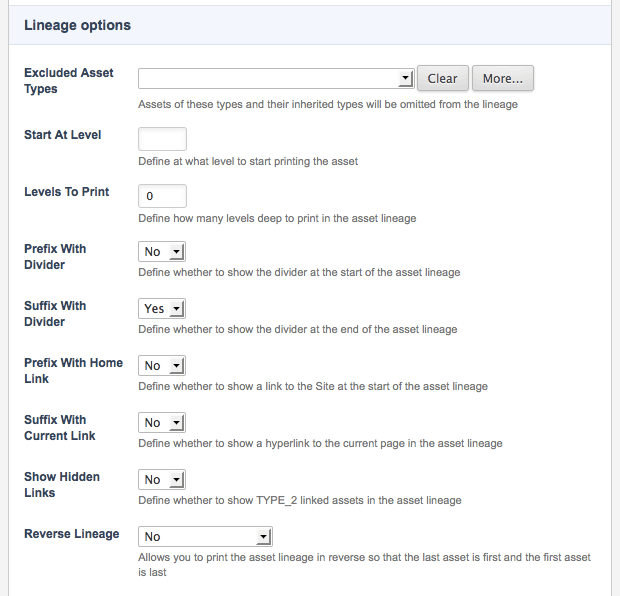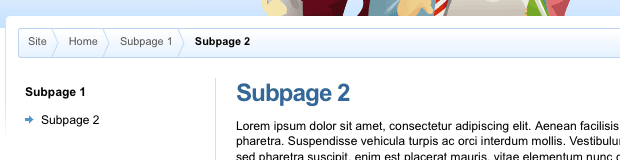# Asset lineage design areaThis design area is used to display the user’s current position in the hierarchy of the site. Matrix builds the asset lineage based on where the asset is located in your site. If the asset has been linked to multiple locations, it will have a different asset lineage for each location. For example, in this figure, the Matrix page is linked under squiz labs and products. The asset lineage for the Matrix page will be   Squiz Labs or   Squiz Products, depending on how the user navigated to the page.

An example of an asset lineage design area is shown:

``````<MySource_AREA id_name="page_lineage" design_area="asset_lineage">
<MySource_SET name="prefix_with_divider" value="true" />
<MySource_SET name="suffix_with_divider" value="false" />
<MySource_DIVIDER>
<img src="files/arrow.jpg" border="0" alt="divider image" width="12" height="8">&nbsp;
</MySource_DIVIDER>
<MySource_ASSET>
<MySource_PRINT var="asset_short_name" />
</a>
</MySource_ASSET>
</MySource_AREA>``````

## Set variables

The set variables available for the asset lineage design area are as follows:

levels_to_print

This variable allows you to define how many levels deep to print in the asset lineage. For example, say you set this value to `1`. Using the example previously outlined, this will only show About Us instead of   Squiz Labs. If you enter a negative value, the last levels of the lineage are shown. For example, if you set this value to `-1`, the asset lineage will show Squiz Labs. To print all levels, set this to `0` or remove the variable from the parse file. The next example sets the level to print to `5`.

``````<MySource_AREA id_name="page_lineage" design_area="asset_lineage">
<MySource_SET name="levels_to_print" value="5" />
<MySource_ASSET>
<MySource_PRINT var="asset_short_name" />
</a>
</MySource_ASSET>
<MySource_DIVIDER>&nbsp;&gt;&nbsp;</MySource_DIVIDER>
</MySource_AREA>``````
start_at_level

This variable allows you to define at what level to start printing the asset lineage. For example, say you set this value to `2`. Using the example previously outlined, this will show Squiz labs. If you enter a negative value, the last levels of the lineage are shown. For example, if you set this value to `-2`, the asset lineage will show   Squiz Labs. The next example sets the start level to `2`.

``````<MySource_AREA id_name="page_lineage" design_area="asset_lineage">
<MySource_SET name="start_at_level" value="2" />
<MySource_ASSET>
<MySource_PRINT var="asset_short_name" />
</a>
</MySource_ASSET>
<MySource_DIVIDER>&nbsp;&gt;&nbsp;</MySource_DIVIDER>
</MySource_AREA>``````
prefix_with_divider

This variable allows you to define whether to show the divider at the start of the asset lineage. By default, this is set to `false`. The next example sets this value to `true`, meaning that it will be shown.

``````<MySource_AREA id_name="page_lineage" design_area="asset_lineage">
<MySource_SET name="prefix_with_divider" value="true" />
<MySource_ASSET>
<MySource_PRINT var="asset_short_name" />
</a>
</MySource_ASSET>
<MySource_DIVIDER>&nbsp;&gt;&nbsp;</MySource_DIVIDER>
</MySource_AREA>``````
suffix_with_divider

This variable allows you to define whether to show the divider at the end of the asset lineage. By default, this is set to `false`. The next example sets this value to `true`, meaning that it will be shown.

``````<MySource_AREA id_name="page_lineage" design_area="asset_lineage">
<MySource_SET name="suffix_with_divider" value="true" />
<MySource_ASSET>
<MySource_PRINT var="asset_short_name" />
</a>
</MySource_ASSET>
<MySource_DIVIDER>&nbsp;&gt;&nbsp;</MySource_DIVIDER>
</MySource_AREA>``````

This variable defines whether to show a link to the site at the start of the asset lineage. When the user clicks this link, they will be taken to the home page of the site. By default, this is set to `false`. The next example sets this value to `true`, meaning that it will be shown.

``````<MySource_AREA id_name="page_lineage" design_area="asset_lineage">
<MySource_ASSET>
<MySource_PRINT var="asset_short_name" />
</a>
</MySource_ASSET>
<MySource_DIVIDER>&nbsp;&gt;&nbsp;</MySource_DIVIDER>
</MySource_AREA>``````

This variable allows you to define whether to show a hyperlink to the current page in the asset lineage. By default, this is set to `false`. The next example sets this value to `true`, meaning that it will be shown.

``````<MySource_AREA id_name="page_lineage" design_area="asset_lineage">
<MySource_ASSET>
<MySource_PRINT var="asset_short_name" />
</a>
</MySource_ASSET>
<MySource_DIVIDER>&nbsp;&gt;&nbsp;</MySource_DIVIDER>
</MySource_AREA>``````

This variable allows you to define whether to show hidden link linked assets in the asset lineage. By default, this is set to `true`. The next example sets this value to `false`, meaning that only menu link linked assets will be shown.

``````<MySource_AREA id_name="page_lineage" design_area="asset_lineage">
<MySource_ASSET>
<MySource_PRINT var="asset_short_name" />
</a>
</MySource_ASSET>
<MySource_DIVIDER>&nbsp;&gt;&nbsp;</MySource_DIVIDER>
</MySource_AREA>``````
unwanted_asset_types

This variable allows you to define a list of asset types to exclude from the asset lineage. For example, you can hide any folders from the asset lineage. If you want to hide multiple asset types, use a comma-separated list of values. The next example hides the folder asset type from the asset lineage.

``````<MySource_AREA id_name="page_lineage" design_area="asset_lineage">
<MySource_SET name="unwanted_asset_types" value="folder" />
<MySource_ASSET>
<MySource_PRINT var="asset_short_name" />
</a>
</MySource_ASSET>
<MySource_DIVIDER>&nbsp;&gt;&nbsp;</MySource_DIVIDER>
</MySource_AREA>``````
reverse_lineage

This variable allows you to print the asset lineage in reverse so that the last asset is first and the first asset is last. This setting may be useful if you want to print the asset lineage right-aligned instead of left-aligned. The next example set this value to `true`, meaning that it will print in reverse.

``````<MySource_AREA id_name="page_lineage" design_area="asset_lineage">
<MySource_SET name="reverse_lineage" value="true" />
<MySource_ASSET>
<MySource_PRINT var="asset_short_name" />
</a>
</MySource_ASSET>
<MySource_DIVIDER>&nbsp;&gt;&nbsp;</MySource_DIVIDER>
</MySource_AREA>``````

To show information about the asset in the asset lineage, you can use the standard keyword replacements along with the `mysource_print` design tag. For example, if you want to print the asset’s short name along with its URL in the lineage, use the keyword replacements. An example of this is shown in the code:

``````<MySource_AREA id_name="page_lineage" design_area="asset_lineage">
<MySource_ASSET>
<MySource_PRINT var="asset_short_name"/>
</a>
</MySource_ASSET>
<MySource_DIVIDER>&nbsp;&gt;&nbsp;</MySource_DIVIDER>
</MySource_AREA>``````

You can also use the metadata keyword replacement to print information from a metadata field along with the standard keyword replacements.

## Customizing the asset lineage design area

When you create a design customization, you can customize the asset lineage design area and select which asset types to exclude. This customization is done in the lineage options section of the asset lineage design area’s Details screen.To exclude an asset type:

1. In the Excluded asset types field, select the asset type you want to exclude.

2. Click the button to select additional asset types. An additional field will appear on the screen.

Read the Design customizations for more information on creating design customizations and how to customize design areas.

## Example

The HTML code that was added to the static HTML file to show where the asset lineage should go in the design is shown here:

``````<div id="page-content">
<!-- Asset Lineage to go here -->
<div><MySource_PRINT var="asset_short_name" /></div>
</div>``````

The required code for the asset lineage design area is added, as shown:

``````<div id="page-content">
<MySource_SET name="prefix_with_divider" value="false" />
<MySource_SET name="suffix_with_divider" value="true" />